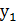### Sample Problem

What is the equation of a line with slope 4 that passes through the point (1,2)?

#### Solution

The point-slope form of an equation is y -.

The slope, m, is 4,is 1, andis 2.

y – 2 = 4(x – 1)

(B) is the correct equation.